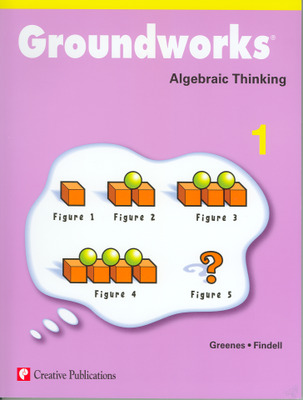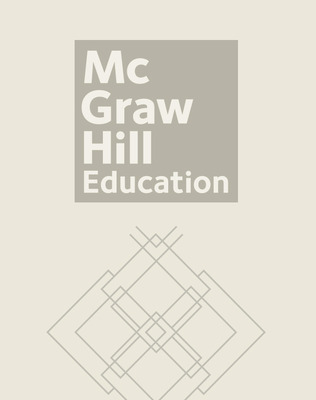# Groundworks Algebraic Thinking

Groundworks: Algebraic Thinking helps students develop their reasoning skills in algebra by providing innovative problems that connect arithmetic and algebra. Each set of problems has a strong emphasis on reasoning and contains ample teacher support. Each title is available for grades 1-7 and contains reproducible pages.Big Ideas

Just like the other titles in the Groundworks series, Groundworks: Algebraic Thinking centers on the big ideas in algebra. Under each big idea, you'll find sets of problems that contain lessons with strong reasoning emphasis. The six big ideas in Groundworks: Algebraic Thinking are:

• Representation
• Proportional Reasoning
• Balance
• Variable
• Function
• Inductive Reasoning

Text Organization

Each text in Groundworks contains 15 different sets of reproducible problem pages. A set refers to a specific type of mathematical reasoning problem. Each set contains six different problems for ample practice reinforcement. With 15 different sets of six problems, each text totals 90 different mathematical reasoning problems.

Problem Sets

Each problem set consists of eight pages and begins with a teaching information page. This page contains several features to help guide the teacher through the activity, either as a class or individually. Following the teacher page are six pages of student problems, each containing one problem, all addressing the same concept. The final page in the problem set is a solutions page.

## Program Components

••Groundworks: Algebra Puzzles and Problems Teacher Resource Book Grade 6
Sort by: